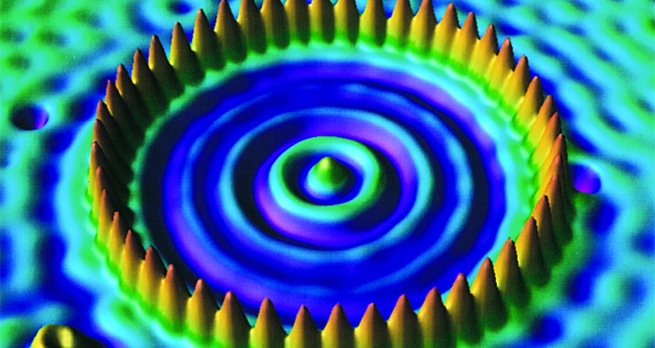Collisions and conservation laws

Start this free course now. Just create an account and sign in. Enrol and complete the course for a free statement of participation or digital badge if available.

Free course

# 3.2 Elastic collisions in general

We now consider the general one-dimensional elastic collision between particles of mass and which move with initial velocities and before the collision and final velocities and after the collision. As stated earlier, the outcome of collisions of this kind is determined by Equation 1 and Equation 2. We shall not write down the details (though you might like to work them out for yourself) but by arguments similar to those used in Example 1, the following result can be obtained.

If the initial velocity of particle relative to particle is taken to be

and if the final velocity of particle relative to particle is taken to be

then, as a result of an elastic collision

In other words:

In a one-dimensional elastic collision between two particles the relative velocity of approach is the negative of the relative velocity of separation

Equation label: (7)

Combining this result (which incorporates the conservation of kinetic energy) with Equation 1 (which expresses conservation of momentum), leads to the following expressions for the final velocities:

Equation label: (8)
Equation label: (9)

In the next two exercises you can use Equations 8 and 9. However, these equations are complicated so you are not expected to memorise them. You should be able to solve this type of question starting from the equations of conservation of momentum (Equation 1) and kinetic energy (Equation 2).

## Activity 3

A neutron of mass rebounds elastically in a head-on collision with a gold nucleus of mass that is initially at rest. What fraction of the neutron’s initial kinetic energy is transferred to the recoiling gold nucleus? Repeat this calculation when the target is a carbon nucleus at rest and of mass .

The initial kinetic energy is

and the final kinetic energy is

therefore the loss in energy is

and the fractional loss is

With in Equation 8,

we can write the fractional loss as

For gold

For carbon

So, a low-mass nucleus is much more effective than a more massive nucleus when it comes to slowing down fast neutrons by elastic collisions. It is because of this fact that carbon is used in a nuclear reactor for just this purpose.

## Activity 4

A tennis player returns a service in the direction of the server. The ball of mass arrives at the racket of mass with a speed of and the racket is travelling at at impact. Calculate the velocity of the returning ball, assuming elastic conditions.

We designate the ball as particle and the racket as particle , with the ball initially travelling along the positive -direction. From Equation 8

so

i.e.

S217_1